# How Geometric Design of Roads Works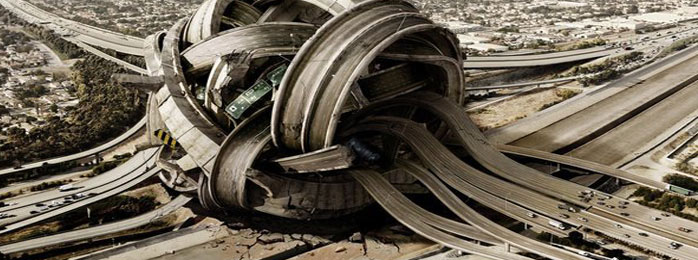Many students have cars nowadays and, surely, going on holiday with friends, they choose the most secure route to their destination. Everybody will agree that it is much more convenient to drive on the “smooth” road with good visibility and without sudden turnings.  Such roads are common now, but they have not always been that way.

The first roads and railways had the form of straight sections connected with circles’ curves.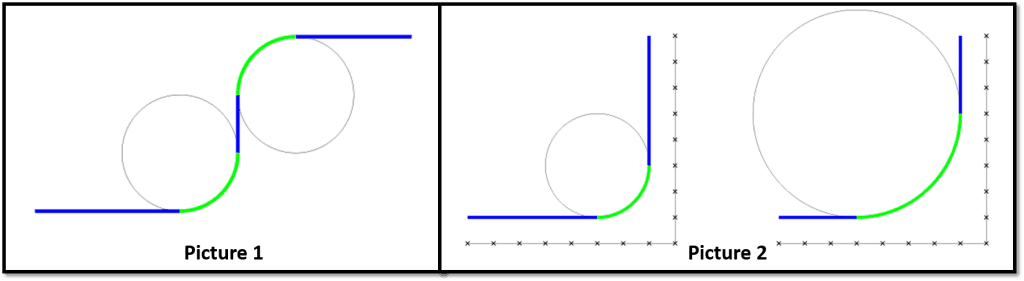In the picture 1 that is above, you see the first schemes of roads. At that time, trains and cars moved slowly and everything seemed perfect. But when the vehicles started gaining higher speed, the sudden tossing appeared in the junctions between the sections when coming into the corner. Therefore, engineers began solving the problem and found the answer in mathematics and physics. In simple terms, they needed the additional transition between the straight line and circle, and here’s why.

Understanding requires the knowledge of the radius of curvature and the centrifugal force. As for the circle, the radius of curvature is simply the radius of this circle. A straight line (can be assumed as a veeery big curve) has the radius of curvature of infinite value. Easy, isn’t it? The force is a ‘mass timed to acceleration’, so simplify it a bit – the centrifugal force has the following formulation

$F = m \cdot \frac {v ^ {2}} {r}$

where $m$ – the mass
$v$ – the speed
$r$ – the radius of curvature.

Pay attention that the force will grow if the mass and the speed  increase. The mass m (mass of the vehicle and its passengers) can be reduced, but you know well that it is not an easy task. It is possible to drive slower, but then it will take more time, so it’s not really comfortable.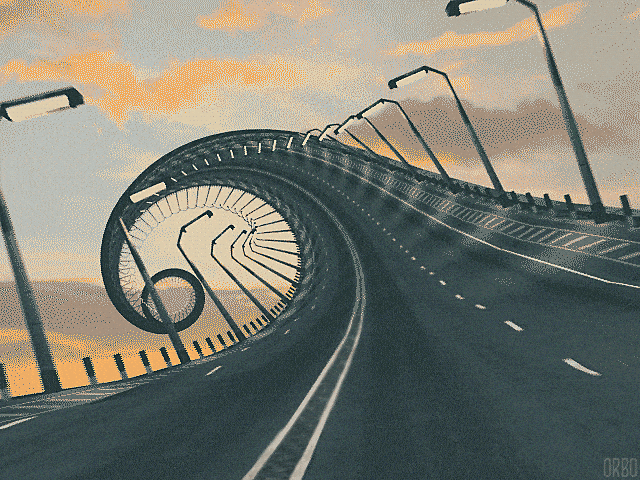On the other hand, we have the radius of curvature which is in the denominator. Thus, increasing the radius, the centrifugal force can be reduced. However, in this case, the line segments become shorter (as in the picture 2) and surely nobody will like it.

The solution is to enter the transition curve between the straight line and the circle, so that the radius of curvature of the line could gradually decrease from infinity (or a big number) to the radius of the circle.  Thus, we can notice the inversely proportional relationship that defines our curve spiral, named Euler’s spiral.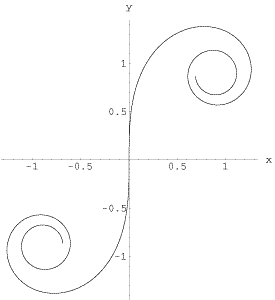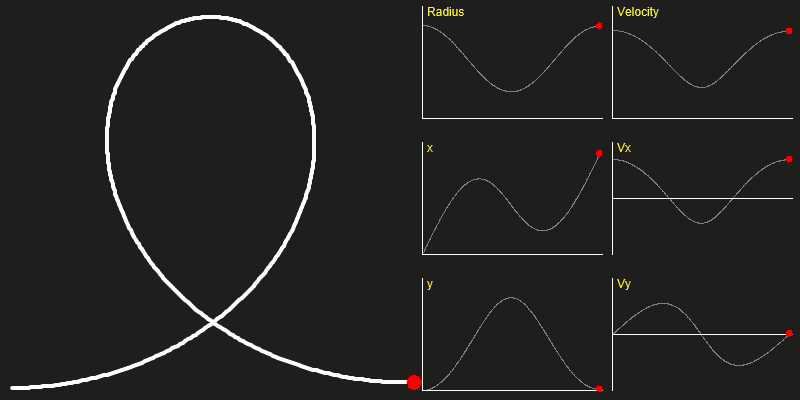So, when you travel by car or by train, you usually move along a straight line – a spiral – a circle – a spiral – a straight line. Thus, the centrifugal force is being slowly changed, and you can turn gradually, instead of doing it sharply.

In addition to the more or less ordinary rails and roads, Euler’s spiral is also used on the racetrack and a roller coaster. So, next time you are on the turns, remember all these math and physics.

30 Shares
Filed under Engineering, Math, Physics.